# Expanded Form - Definition with Examples

The Complete K-5 Math Learning Program Built for Your Child

• 40 Million Kids

Loved by kids and parent worldwide

• 50,000 Schools

Trusted by teachers across schools

• Comprehensive Curriculum

Aligned to Common Core

## Expanded Form Games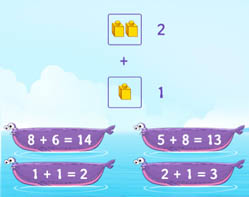Use symbols and numbers to represent addition within 10 as addition sentences like 2 + 3 = 5.

Covers Common Core Curriculum 1.OA.7

## What is Expanded Form?

In the expanded form. we break up a number according to their place value and expand it to show the value of each digit. For example, the expanded form of 943 is given.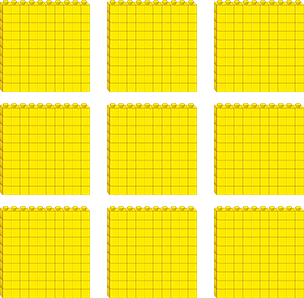9 blocks of hundreds4 blocks of tens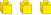3 blocks of ones

9 hundreds 4 tens 3 ones

Expanded Form:    900  +   40   +   3

Each number has a place value. It determines the value of that digit according to its position in the number. The value of a digit in a number increases as we move from left to right. The digits on the left have lower place value than the digits on the right.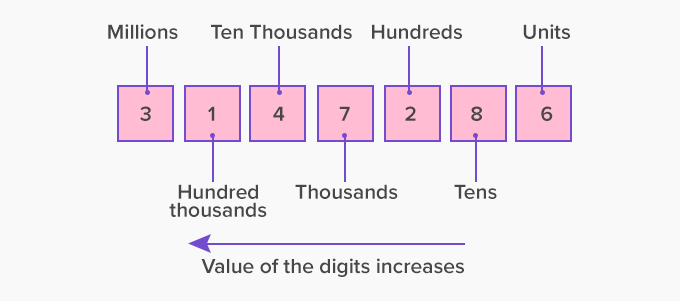To write the number 7286 in expanded form we follow the given steps:

Step 1: Use place value diagram to express the number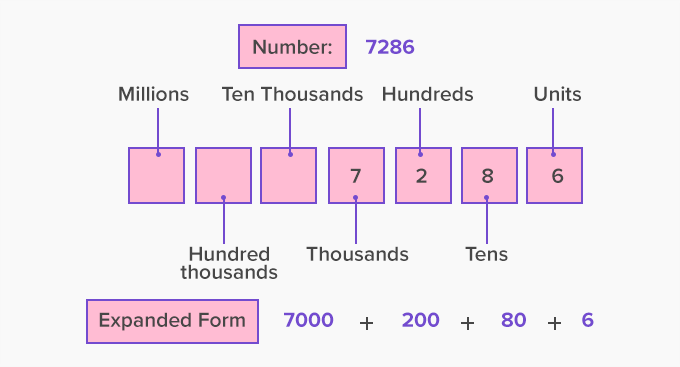Step 2: Write the number in expanded form.

7286 = 7000 + 200 + 80 + 6

Expanded form of decimal numbers

The decimal digits also have place values. A decimal number can be expanded as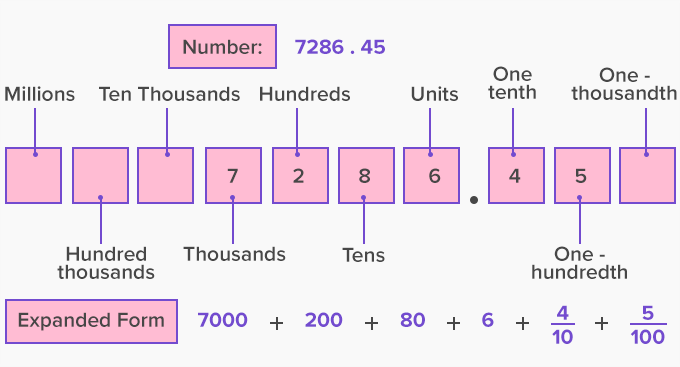Fun Facts Expanded form is not same as expanded notation.  The expanded notation a number is represented as the sum of each digit in a number multiplied by its place value.  Expanded form: 234 = 200 + 30 + 4 Expanded notation: 234 = (2  100) + (3  10) + (4  1) In the expanded forms, we use only addition between the place value numbers and in the expanded notation we use addition and multiplication.

Won Numerous Awards & Honors